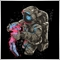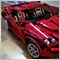# Advanced: Close All Trades at Daily Profit Target90

Hi guys!

I have been working on an EA for a few weeks now and I am stuck: I want to close all trades and open orders when a profit target (say 10 pips) is reached.

I have a ClosePosition function which doesn't seem to be working.

Would anyone have any idea how to solve this?

Thanks a lot,

Martin

```//+------------------------------------------------------------------+

// So far: first two open order are placed

// ADDING: Close all trades and orders if overall profit is 10 pips

//+------------------------------------------------------------------+
#property copyright "Copyright 2019, Martin Ellul."

#include <Trade\Trade.mqh>
#include <Trade\SymbolInfo.mqh>
#include <Trade\PositionInfo.mqh>
#include <Trade\AccountInfo.mqh>

input double PositionSize                       = 1;      // Position size (cost of one pip)
input double PositionHedge                      = 3;      // Size of hedge position
input int StopLossDistance                      = 30;     // Stop loss distance in pips
input bool IsUseTrailingStop                    = false;  // Use trailing stop
input int  TrailingStopDistance                 = 40;     // Trailing stop distance in pips
input bool UseTakeProfit                        = false;   // Use Take Profit
input int  Take_Profit                          = 30;     // Take Profit
input int RSIuplimit                            = 70;     // RSI Upper Limit
input int RSIlowlimit                           = 30;     // RSI Lower Limit
input double AccountProfit                      = 100;    // Account Profit

string HoursandMinutes;
int Previouscount;
double currentBid, currentAsk;
double morningbid, morningask;
bool   ext_hedging=true;
CTrade ext_trade;
CTrade trade;
CSymbolInfo symbol_info;
ulong arr_ticket_long_rsi[];
ulong arr_ticket_short_rsi[];
double adjusted_point;
double trailing_stop_value;
double stop_loss_value;
double take_profit_value;
int digits_adjust=1;
int tick_counter=0;
int time_in_open_function=-1;
int time_in_close_function=-1;
double Long1, Long2, Short1, Short2;

#define MA_MAGIC 2719281928459450

//+------------------------------------------------------------------+
//| Expert initialization function                                   |
//+------------------------------------------------------------------+
int OnInit()
{

//--- prepare trade class to control positions if hedging mode is active
ext_hedging=((ENUM_ACCOUNT_MARGIN_MODE)AccountInfoInteger(ACCOUNT_MARGIN_MODE)==ACCOUNT_MARGIN_MODE_RETAIL_HEDGING);
ext_trade.SetExpertMagicNumber(MA_MAGIC);
ext_trade.SetMarginMode();
symbol_info.Name(_Symbol);

if(symbol_info.Digits()==3 || symbol_info.Digits()==5)
digits_adjust=10;
adjusted_point=symbol_info.Point()*digits_adjust;
trailing_stop_value=TrailingStopDistance*adjusted_point;
stop_loss_value=StopLossDistance*adjusted_point;
take_profit_value=Take_Profit*adjusted_point;

ArrayResize(arr_ticket_long_rsi,0,100000);
ArrayResize(arr_ticket_short_rsi,0,100000);

return(INIT_SUCCEEDED);
}
//+------------------------------------------------------------------+
//| Expert deinitialization function                                 |
//+------------------------------------------------------------------+
void OnDeinit(const int reason)
{

}

//+------------------------------------------------------------------+
//| Expert tick function                                             |
//+------------------------------------------------------------------+
void OnTick()
{

// get local time
datetime time= TimeLocal();
//format time and create a string
HoursandMinutes = TimeToString(time,TIME_MINUTES);

currentBid = SymbolInfoDouble(_Symbol,SYMBOL_BID); // Get latest Bid Price
currentAsk = SymbolInfoDouble(_Symbol,SYMBOL_ASK); // Get latest Ask Price

//  CheckForClose();
CheckForOpen();

}
//+------------------------------------------------------------------+
//| Try to open the deal                                          |
//+------------------------------------------------------------------+
void CheckForOpen()

{
MqlRates rt;
if(CopyRates(_Symbol,_Period,0,2,rt)!=2)
{
Print("CopyRates of ",_Symbol," failed, no history");
return ;
}

datetime cur_time=rt.time;
MqlDateTime mdt;
TimeToStruct(cur_time,mdt);
int cur_calculated_time=mdt.hour*60+mdt.min;
if(cur_calculated_time==time_in_open_function)
return;
time_in_open_function=cur_calculated_time;

//remove unnecessary orders
RefreshOrders();
//move trailing stop orders if it is necessary
ModifyOrders();

string str_date_time= TimeToString(TimeCurrent())+" ";

// IF DAILYPROFIT IS < 100) THEN PROCEED

// Place Open Order at 07:55 UK time
if (StringSubstr(HoursandMinutes,0,5)=="07:55")
{
morningbid = SymbolInfoDouble(_Symbol,SYMBOL_BID); // Get bid price at 07:55am UK time
morningask = SymbolInfoDouble(_Symbol,SYMBOL_ASK); // Get ask price at 07:55am UK time

Long1  = morningask + 3;
Short1 = morningbid - 3;

trade.BuyStop(1, Long1,_Symbol,0,Long1 + 10,ORDER_TIME_GTC,TimeCurrent()+3600,0);
trade.SellStop(1,Short1,_Symbol,0,Short1 - 10,ORDER_TIME_GTC,TimeCurrent()+3600,0);

}

// if only 1 position is open then proceed

if (OrdersTotal() < 1);
{

ENUM_POSITION_TYPE firstlong=PositionGetInteger(POSITION_TYPE);
double open_price_firstlong=PositionGetDouble(POSITION_PRICE_OPEN);
double current_stop_loss_firstlong=PositionGetDouble(POSITION_SL);
double local_stop_loss_firstlong;

if (firstlong == POSITION_TYPE_BUY)
{

trade.SellStop(2,Short1,_Symbol,0,Short1 - 8,ORDER_TIME_GTC,TimeCurrent()+3600,0);

}

ENUM_POSITION_TYPE firstshort=PositionGetInteger(POSITION_TYPE);
double open_price_firstshort=PositionGetDouble(POSITION_PRICE_OPEN);
double current_stop_loss_firstshort=PositionGetDouble(POSITION_SL);
double local_stop_loss_firstshort;

if (firstshort == POSITION_TYPE_SELL)
{

trade.BuyStop(2, Long1,_Symbol,0,Long1 + 8,ORDER_TIME_GTC,TimeCurrent()+3600,0);

}
}

}
//+------------------------------------------------------------------+
//| Open position                                                  |
//+------------------------------------------------------------------+
void OpenPosition(ulong& arr_ticket[],ENUM_ORDER_TYPE order_type)
{

if(TerminalInfoInteger(TERMINAL_TRADE_ALLOWED) && Bars(_Symbol,_Period)>100)
{
string str_date_time= TimeToString(TimeCurrent())+" ";
double stop_loss=0;
double take_profit=0;
double local_stop_loss_value=IsUseTrailingStop ? trailing_stop_value:stop_loss_value;

//        double local_take_profit_value= UseTakeProfit ? take_profit_value:take_profit_value;
double ask_price=SymbolInfoDouble(_Symbol,SYMBOL_ASK);
double bid_price=SymbolInfoDouble(_Symbol,SYMBOL_BID);
//Calculate stop loss price
if(local_stop_loss_value!=0)
stop_loss=order_type==ORDER_TYPE_SELL ? NormalizeDouble(ask_price+local_stop_loss_value,Digits()):
NormalizeDouble(bid_price-local_stop_loss_value,Digits());

// Calculating take profit price
//        if(local_take_profit_value!=0)
take_profit=order_type==ORDER_TYPE_SELL ? NormalizeDouble(bid_price-take_profit_value,Digits()):
NormalizeDouble(ask_price+take_profit_value,Digits());

//           take_profit=order_type==ORDER_TYPE_SELL ? NormalizeDouble(ask_price-local_take_profit_value,Digits()):
//                                                       NormalizeDouble(bid_price+local_take_profit_value,Digits());

//Calculate lot size
double lot_size=GetLotSize();
string str_comment=_Symbol+_Period+TimeToString(TimeCurrent());
bool is_open=ext_trade.PositionOpen(_Symbol,order_type,lot_size,
SymbolInfoDouble(_Symbol,order_type==ORDER_TYPE_SELL ? SYMBOL_BID:SYMBOL_ASK),
stop_loss,take_profit,str_comment);
uint result_ret_code=ext_trade.ResultRetcode();
if(is_open)
{
Print("Terminal opened the deal for ",_Symbol);
ulong ticket=ext_trade.ResultDeal();
uint total=PositionsTotal();
for(uint i=0; i<total; i++)
{
string symbol=PositionGetSymbol(i);
string comment=PositionGetString(POSITION_COMMENT);
if(symbol==_Symbol && comment==str_comment)
{
ticket=PositionGetInteger(POSITION_TICKET);

}

}
int array_size=ArraySize(arr_ticket);
ArrayResize(arr_ticket,array_size+1);
arr_ticket[array_size]=ticket;

}
else
{
Print("Terminal could not open deal for ",_Symbol);
Print("Terminal error code= ",result_ret_code);
}
}

}

//+------------------------------------------------------------------+
//| Close position with the specified ticket                                                 |
//+------------------------------------------------------------------+
void ClosePosition(ulong& arr_tickets[])
{
string date_time_string=TimeToString(TimeCurrent())+" ";
for(int i=0;i<ArraySize(arr_tickets);i++)
{
ulong ticket=arr_tickets[i];

if (AccountInfoDouble(ACCOUNT_PROFIT) == AccountProfit)
{

bool is_selected=PositionSelectByTicket(ticket);
if(is_selected)
{

if(TerminalInfoInteger(TERMINAL_TRADE_ALLOWED) && Bars(_Symbol,_Period)>100)
{
bool is_close=ext_trade.PositionClose(ticket,3);
if(!is_close)
{
uint result_ret_code=ext_trade.ResultRetcode();
Print("Terminal could not close the position for",_Symbol," the error Code=",result_ret_code);
}
}

}
}
else
{

}
ticket=0;

}
ArrayResize(arr_tickets,0,10000);
}
//+------------------------------------------------------------------+
//| Remove unnecessary orders from tickets array                                                 |
//+------------------------------------------------------------------+
void RefreshArray(ulong& arr_ticket[])
{
ulong buffer_array[];
ArrayResize(buffer_array,0,10000);
int count=0;
for(int i=0;i<ArraySize(arr_ticket);i++)
{
ulong ticket=arr_ticket[i];
bool is_selected=PositionSelectByTicket(ticket);
if(is_selected)
{
ArrayResize(buffer_array,count+1,10000);
buffer_array[count]=ticket;
count++;
}
}
if(ArraySize(buffer_array)!=ArraySize(arr_ticket))
{
ArrayResize(arr_ticket,ArraySize(buffer_array),10000);
ArrayCopy(arr_ticket,buffer_array,0,0,WHOLE_ARRAY);
}
}
//+------------------------------------------------------------------+
//| Remove unnecessary orders from all tickets array                                                 |
//+------------------------------------------------------------------+
void RefreshOrders()
{
RefreshArray(arr_ticket_long_rsi);
RefreshArray(arr_ticket_short_rsi);
}
//+------------------------------------------------------------------+
//| Get lot size for the deal                                                 |
//+------------------------------------------------------------------+
double GetLotSize()
{
double lot_step=SymbolInfoDouble(_Symbol,SYMBOL_VOLUME_STEP);
double lot_value=SymbolInfoDouble(_Symbol,SYMBOL_TRADE_TICK_VALUE);
double min_lot=SymbolInfoDouble(_Symbol,SYMBOL_VOLUME_MIN);
double max_lot=SymbolInfoDouble(_Symbol,SYMBOL_VOLUME_MAX);
double lot_size_for_deal=1.0*PositionSize/(lot_value*digits_adjust);
lot_size_for_deal=GetLotSize(lot_size_for_deal, lot_step,min_lot,max_lot);
return lot_size_for_deal;
}
//+------------------------------------------------------------------+
//| Get best fit lot size for the calculated one
//+------------------------------------------------------------------+
double GetLotSize(double calculated_lot_size,double lot_size_step,double min_lot_size,double max_lot_size)
{
if(calculated_lot_size<min_lot_size)
return min_lot_size;
if(calculated_lot_size>max_lot_size)
return max_lot_size;
double cur_lot_size=lot_size_step;
double next_lot_size=cur_lot_size+lot_size_step;
do
{
if(calculated_lot_size>=cur_lot_size && calculated_lot_size<=next_lot_size)
{
if(calculated_lot_size==cur_lot_size)
return cur_lot_size;
if(calculated_lot_size==next_lot_size)
return next_lot_size;
if(calculated_lot_size-cur_lot_size<next_lot_size-calculated_lot_size)
return cur_lot_size;
else
return next_lot_size;
}
cur_lot_size=next_lot_size;
next_lot_size=cur_lot_size+lot_size_step;
}while(true);
}
//+------------------------------------------------------------------+
//| Move trailing stop if it is necessary for all orders                                                 |
//+------------------------------------------------------------------+
void ModifyOrders()
{
ModifyOrders(arr_ticket_long_rsi);
ModifyOrders(arr_ticket_short_rsi);
}
//+------------------------------------------------------------------+
//| Move trailing stop if it is necessary for all orders from array                                                |
//+------------------------------------------------------------------+
void ModifyOrders(ulong& arr_ticket[])
{

if(!IsUseTrailingStop || TrailingStopDistance==0)
return;
for(int i=0;i<ArraySize(arr_ticket);i++)
{
ulong ticket=arr_ticket[i];
bool is_selected=PositionSelectByTicket(ticket);
if(is_selected)
{
ENUM_POSITION_TYPE position_type=PositionGetInteger(POSITION_TYPE);
double open_price=PositionGetDouble(POSITION_PRICE_OPEN);
double current_stop_loss=PositionGetDouble(POSITION_SL);
double local_stop_loss;
double ask_price=SymbolInfoDouble(_Symbol,SYMBOL_ASK);
double bid_price=SymbolInfoDouble(_Symbol,SYMBOL_BID);
if(position_type==POSITION_TYPE_BUY)
{
if(bid_price-open_price>trailing_stop_value)
{
local_stop_loss=NormalizeDouble(bid_price-trailing_stop_value,Digits());
if(current_stop_loss<local_stop_loss)
{
ext_trade.PositionModify(ticket,local_stop_loss,0);
}
}
}
else if(position_type==POSITION_TYPE_SELL)
{
if(open_price-ask_price>trailing_stop_value)
{
local_stop_loss=NormalizeDouble(ask_price+trailing_stop_value,Digits());
if(current_stop_loss>local_stop_loss)
{
ext_trade.PositionModify(ticket,local_stop_loss,0);
}

}
}

}
}

}
```425

Martin:

I have been working on an EA for a few weeks now and I am stuck: I want to close all trades and open orders when a profit target (say 10 pips) is reached.

I have a ClosePosition function which doesn't seem to be working.

Would anyone have any idea how to solve this?

Before we even look at close, your EA won't even open... Check these lines:

```   morningbid = SymbolInfoDouble(_Symbol,SYMBOL_BID); // Get bid price at 07:55am UK time
morningask = SymbolInfoDouble(_Symbol,SYMBOL_ASK); // Get ask price at 07:55am UK time

Long1  = morningask + _Point*3;
Short1 = morningbid - _Point*3;
```

You cannot just add 3 to a price... you need to multiply it to _Point first. And if your broker uses 3 or 5 digits (check _Digits), which is highly probably nowadays, you should multiple _Point by 30 instead of 3.

And where do you plan to call ClosePosition function? (it isn't called at all, so of course it doesn't work).90

Seng Joo Thio:

Before we even look at close, your EA won't even open... Check these lines:

You cannot just add 3 to a price... you need to multiply it to _Point first. And if your broker uses 3 or 5 digits (check _Digits), which is highly probably nowadays, you should multiple _Point by 30 instead of 3.

And where do you plan to call ClosePosition function? (it isn't called at all, so of course it doesn't work).

Thanks for the tip on adding 3 pips, I hadn't seen that.

I'm calling it in OnTick, at the same place as CheckforOpen, as CheckForClose().

I removed the if statement (below) from the ClosePosition function and added it in this new function which should close all positions.

```void CheckForClose()
{

if (AccountInfoDouble(ACCOUNT_PROFIT) == AccountProfit)
{

ClosePosition(arr_ticket_long_rsi);
ClosePosition(arr_ticket_short_rsi);

}

} ```425

Martin:

Thanks for the tip on adding 3 pips, I hadn't seen that.

I'm calling it in OnTick, at the same place as CheckforOpen, as CheckForClose().

I removed the if statement (below) from the ClosePosition function and added it in this new function which should close all positions.

A number of issues:

1. In your CheckForClose(), you cannot compare floating numbers with ==, because they're rarely equal. Should change to >= instead.
2. Your arr_ticket_long_rsi and arr_ticket_short_rsi arrays - you iterate through them in ClosePositions(), RefreshArray() and ModifyOrders(), but they were never filled with any data. That explains why your positions are never closed (and not modified too).
3. Long1 and Short1 - only set at specific time. Beyond that time, your code still attempt to set buystop and sell stop, so you see many error messages when actual price is below Short1 and you try to set sellstop, and vice versa for buystop.
Try fixing these first and see how it performs...
To add comments, please log in or register